# SAT II Math II : Slope

## Example Questions

← Previous 1

### Example Question #1 : Slope

What is the slopeof the line between the points (-1,0)  and (3,5)?

Possible Answers: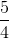Correct answer:Explanation:

For this problem we will need to use the slope equation: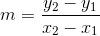In our case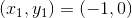and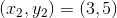Therefore, our slope equation would read: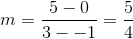### Example Question #1 : Slope

What is the slope of the function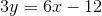Possible Answers:

4

3

6

2

Correct answer:

2

Explanation:

To find the slope of this function we first need to get it into slope-intercept formwhere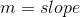To do this we need to divide the function by 3: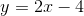From here we can see our m, which is our slope equals 2

### Example Question #3 : Slope

What is the slope for the line having the following points: (1, 5), (2, 8), and (3, 11)?

Possible Answers:

2

4

3

5

Correct answer:

3

Explanation:

To find the slope for the line that has these points we will use the slope formula with two of the points.

In our case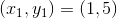and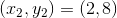Now we can use the slope formula: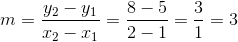### Example Question #4 : Slope

What is the slope of the function: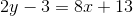Possible Answers:

4

2

8

3

Correct answer:

4

Explanation:

For this question we need to get the function into slope intercept form first which iswhere the m equals our slope.

In our case we need to do algebraic opperations to get it into the desired form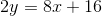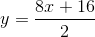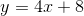Therefore our slope is 4

### Example Question #5 : Slope

Find the slope of the following equation: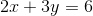Possible Answers: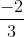Correct answer:Explanation:

To find the slope for a given equation, it needs to first be put into the "y=mx+b" format. Then our slope is the number in front of the x, or the "m". For this equation this looks as follows:

First subtract 2x from both sides: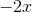That gives us the following: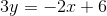Divide all three terms by three to get "y" by itself: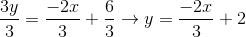This means our "m" is -2/3

### Example Question #6 : Slope

Find the slope of the following equation: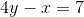Possible Answers: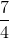Correct answer:Explanation:

To find the slope for a given equation, it needs to first be put into the "y=mx+b" format. Then our slope is the number in front of the x, or the "m". For this equation this looks as follows:

First add x to both sides: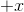That gives us the following: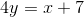Divide all three terms by four to get "y" by itself: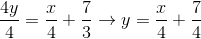This means our "m" is 1/4

### Example Question #7 : Slope

Find the slope of the following equation: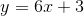Possible Answers:Correct answer:Explanation:

To find the slope for a given equation, it needs to first be put into the "y=mx+b" format. Then our slope is the number in front of the x, or the "m". For this equation this looks as follows:Our equation is already in the "y=mx+b" format, so our "m" is 6.

### Example Question #8 : Slope

Find the slope of the following equation: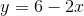Possible Answers:Correct answer:Explanation:

To find the slope for a given equation, it needs to first be put into the "y=mx+b" format. Then our slope is the number in front of the x, or the "m". For this equation this looks as follows:To put our equation in the "y=mx+b" format, flip the two terms on the right side of the equation: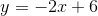So our "m" in this case is -2.

### Example Question #9 : Slope

Find the slope given the equation: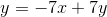Possible Answers: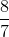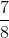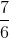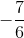Correct answer:Explanation:

Subtract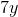on both sides.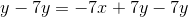Simplify both sides.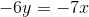Divide by negative 6 on both sides.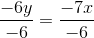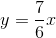The slope is:### Example Question #10 : Slope

Find the slope of the equation: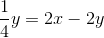Possible Answers: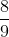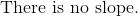Correct answer:Explanation:

To determine the slope, we need the equation in slope intercept form.Multiply by four on both sides to eliminate the fraction.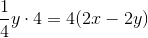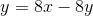Add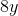on both sides.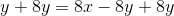Combine like-terms.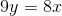Divide by nine on both sides.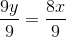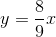The value of, or the slope, is.

← Previous 1

### All SAT II Math II Resources